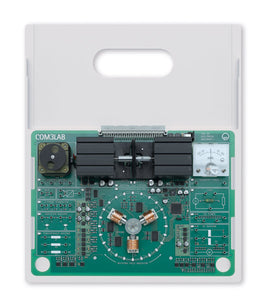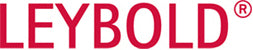Leybold LD Didactic

# 7002501 COM3LAB Course: Electrical Machines I

Regular price \$4,735.00 \$0.00 Unit price per

# COM3LAB Course: Electrical Machines I

Comprises an experiment board, housed within a board carrier, for use in conjunction with, and powered by the Master Unit (700020, supplied separately) teaching a course concerning the connection methods and the recording of characteristics of electric motors and generators, experiments being wired by using 2 mm safety cables (700022, supplied separately). With the integrated machine test system, a multitude of experiments can be carried out, for example the recording of torque, power and current-locus curves. Course content, experiment instructions and tasks are taught via course-specific software

Curriculum Coverage

• Forces in a magnetic field
• Measurement of Lorentz force
• Drives
• Torque-speed characteristics
• Machine test system
• d.c. machines
• Speed and induced voltage with d.c. machines
• Torque and armature voltage with d.c. machines
• Torque and excitation voltage with d.c. machines
• d.c. machines with external excitation
• Reversible of direction
• Characteristics with variable armature voltage
• Characteristics with variable excitation voltage
• Shunt-wound d.c. machines
• Characteristics with variable operating voltage
• Series-wound d.c. machines
• Reversal of direction with d.c. machines
• Characteristics with variable operating voltage
• Generator operation of d.c. machines
• Drive and generator with a resistive load
• Power output of generator
• Rotating field (three-phase) machines
• Three-phase windings
• Rotating fields
• Direction of rotation with periodic swapping of phase conductors
• Direction of rotation when phase conductors are swapped
• Voltage and current in star (Y) circuits
• Voltage and current in delta circuits
• Resistance in stator winding
• Reactance of an a.c. winding
• Synchronous machines
• Equivalent circuit diagram for synchronous machines & how they are used
• Permanently excited synchronous machines
• Step operation of synchronous machines
• Determining rotor position in star configuration
• Determining rotor position in delta configuration
• Synchronous machine at variable speed (run-up)
• Speed measurement
• Speed setting using frequency converter
• Asynchronous machines
• Block and equivalent circuit diagrams for asynchronous machines
• Determination of slip
• Star-delta starting
• Measurement of torque and line currents during run-up
• Changing direction of asynchronous machines
• Recording of torque-speed characteristic for asynchronous machines
• Three-phase drives
• Changing speed of asynchronous machines
• How speed depends on slip
• How speed depends on stator frequency
• Stepper motors
• Full-step operation
• Half-step operation
• Changing the direction of a stepper motor
Virtual Laboratory
• Multi-channel Oscilloscope
• Oscilloscope
• Function Generator
• Multimeter (x2)
• Digital analyser
• Word Processing
• Printer
• Pocket Calculator
• Free Experimentation
• Glossary
The COM3LAB software contains courses in all supported languages and the current version can be downloaded from WWW.LD-DIDACTIC.DE for free. Alternatively, the COM3LAB software can also be obtained on DVD (70000CBT).

Technical Data:

• Synchronous machine with optical rotary field indicator and strobe for speed determination
• Induction motor with star-delta switch
• Optical tachogenerator
• DC motor
• DC generator
• Current-torque converter
• Thermometer
• Three phase generator, phase voltage: 0 V. .. 10 V
• Frequency converter, Frequency: 1 Hz - 80 Hz
• DC supplies
• Stepper motor
• Electrodynamic force meter
• Multichannel oscilloscope